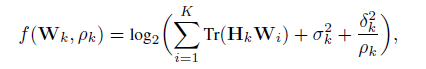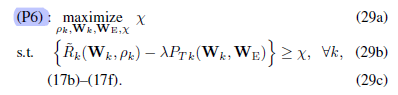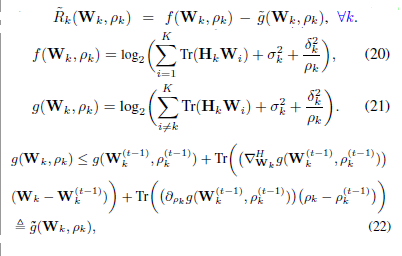# Problem with Illegal operation: log( {convex} )

Hello
I am new in cvx and must simulate a paper which solve a problem by cvx. However there is a problem with thiswhere W_i and 0<ro_k<1 are cvx variables. The problem is this error
Illegal operation: log( {convex} ) that means the argument of log must be concave but I dint know how solve it?!
Thanks in advance if anyone can help me.

You can adapt the approach in How to express log(sum(xi)) in CVX . I leave you to work out the details - that way, you’ll learn something in the process. Divide the log_sum_exp by log(2) to account for use of log2.

Edit: Per my post below, I withdraw this answer.

Dear sir
Thanks a lot for your reply. I followed the attached link but I couldn’t use it. In fact the main problem is as belowwhereWe know that in (29b) that g_tild, P_TK and X are affine expression (W_i and 0<ro_k<1 are variables in this constraint) and lambda is a constant, so function f must be concave although its argument is convex.
I really appreciate if you explain more.

I think my solution was not correct. It required log(affine) as the first term of log_sum_exp, and that is not allowed. So now, I am not even sure that is a convex constraint. Maybe someone else has more definitive answer.

Well, it appears ot be neither convex nor concave. For instance, there is one negative and one positive eigenvalue of the Hessian of `log(y+1/x)` evaluated at x = y = 1. This is a special case of your function.